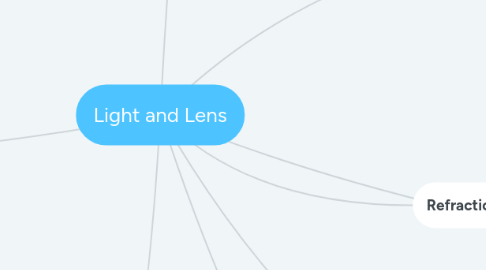# Light and Lens

O Level Physics Light and Lens

Get Started. It's FreeLight and Lens## 1. cause by change of speed of light

### 1.1. n = c/v

1.1.1. use to calculate speed of light in medium

1.1.2. c = 3.0 x 10^8 m/s

1.1.3. n has no unit

1.1.4. n of air or vacuum = 1

## 6. Reflection

### 6.2. Ray Diagram

6.2.1. Locate Virtual Img by Img Dist = Obj DIst

6.2.1.1. Dist is Perpendicular to Mirror Line

6.2.2. Point of intersection between virtual ray and mirror line IS THE point of reflection

6.2.3. Draw virtual ray from img to observer

6.2.4. Add in solid lines and arrows

## 7. Refraction

### 7.1. Snell's law

7.1.1. n sin A = n sin B

7.1.1.1. to get critical angle, set angle in the lower n to 90 degrees

7.1.1.2. Check calculator in degree mode

7.1.1.3. light trapped in higher n medium

7.1.2. n of any other medium MUST > 1

### 7.2. TIR

7.2.1. happen only if

7.2.1.1. angles are measured from ray to normal

7.2.1.2. light travel from higher n medium to lower n

7.2.1.3. angle i > critical angle

7.2.2. Application

7.2.2.1. optical fibre

7.2.2.2. diamond

## 8. Converging Lens

### 8.2. Trick

8.2.1. 1/O + 1/I = 1/f

### 8.3. The object distance determine the type of image produced

8.3.1. OD < 1F

8.3.1.1. V, U, E

8.3.2. OD = 1F

8.3.2.1. no convergence

8.3.3. OD between 1F and 2F

8.3.3.1. R, I, D

8.3.4. OD = 2F

8.3.4.1. R, I, same size

8.3.5. OD > 2F

8.3.5.1. R, I, D

### 8.4. Ray Diagram

8.4.1. Tip to Optical Center

8.4.2. Tip - Horizontal Line - Lens - Focal Point

8.4.3. Parallel Ray - Lens - Some where along Focal Plane

8.4.3.1. Damaged Lens only affect Brightness of Image. No change to ID.